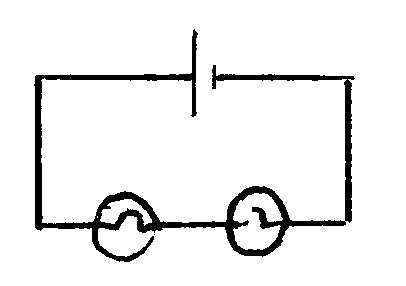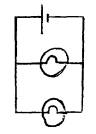MAGNETISM & ELECTRICITY

Use these questions to help you revise.  Move the mouse over the hidden text in the answer box to see the example answers

 QUESTIONS ANSWER BOX 1 . What do we call each end of a magnet? The poles (North and South). 2. Which of these materials are magnetic; copper, paper, iron, glass, steel? Iron and steel, 3. How can you make two magnets repel each other? Put two North poles together or two South poles together. 4. How can you make an electromagnet? Connect a coil of wire to a battery, and pass electricity through the coil. 5. Name 5 uses of electromagnets. In scrapyards, electric motors, door bells, TV sets and loudspeakers 6. What things do you need to find a magnetic field around a magnet? A plotting compass or iron filings. 7. For an electric current to flow we must have a complete _______with no ________ in it. circuit, gaps. 8. What do we call materials which, a) let electricity pass through them, and a) conductors, b) don't let electricity pass through them? b) insulators. 9. Draw two circuits to show the difference between series and parallel circuits. Use one cell and two bulbs in each circuit. SeriesParallel:10. We think that tiny particles move through a wire when electric current flows. What are these particles called? Electrons. 11. What happens to a thin piece of wire when electricity flows through it? It heats up. 12. What do we use to measure the size of an An ammeter. electric current? 13. What units do we use when measuring electric currents? Amperes (amps or A). 14. What do we call the safety devices used in A fuse It breaks (melts) when too much current plugs? How does it work? flows through it. This breaks the circuit. 15. What do we use to measure the size of the 'push' of a battery? A voltmeter. 16. How, do we describe a battery that has lost all of its 'push' ? Flat. 17. Modern houses don't have fuse boxes in their mains circuits. What do they use instead? Circuit breakers.

Some online quizzes to have a go at:

Electrical keywords
Circuit symbols
series circuits
parallel circuits